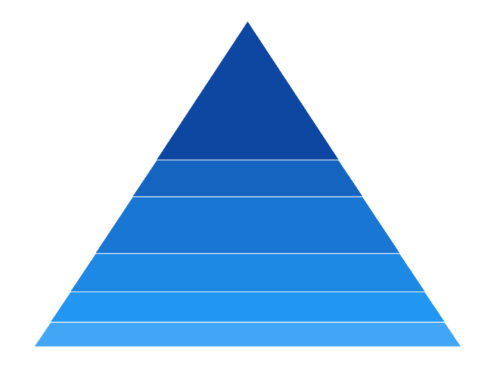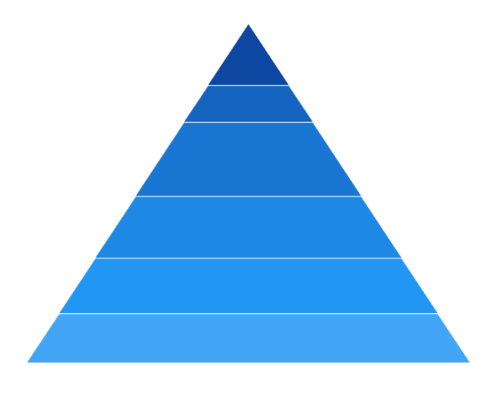# Rendering Mode in WinUI Chart (SfPyramidChart)

15 Sep 20222 minutes to read

The Mode property is used to define the rendering mode of pyramid chart such as Surface or Linear. The default value of Mode property is `Linear`.

## Surface Mode

The Surface mode is used to rendering the area of pyramid chart segments based on data point values.

``````<chart:SfPyramidChart x:Name="chart"
ItemsSource="{Binding Data}"
XBindingPath="Category"
YBindingPath="Value"
Mode="Surface">
</chart:SfPyramidChart>``````
``````SfPyramidChart chart = new SfPyramidChart();
chart.SetBinding(SfPyramidChart.ItemsSourceProperty, new Binding() { Path = new PropertyPath("Data") });
chart.XBindingPath = "Category";
chart.YBindingPath = "Value";
chart.Mode = ChartPyramidMode.Surface;
. . .
this.Content = chart;``````## Linear Mode

The Linear mode is used to rendering the height of pyramid chart segments based on data point values.

``````<chart:SfPyramidChart x:Name="chart"
ItemsSource="{Binding Data}"
XBindingPath="Category"
YBindingPath="Value"
Mode="Linear">
</chart:SfPyramidChart>``````
``````SfPyramidChart chart = new SfPyramidChart();
chart.SetBinding(SfPyramidChart.ItemsSourceProperty, new Binding() { Path = new PropertyPath("Data") });
chart.XBindingPath = "Category";
chart.YBindingPath = "Value";
chart.Mode = ChartPyramidMode.Linear;
. . .
this.Content = chart;``````